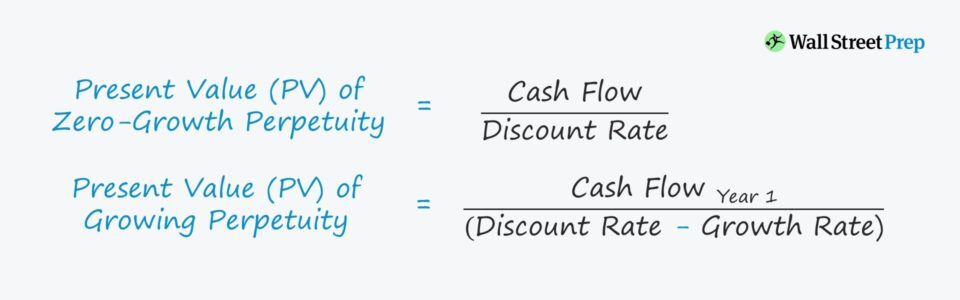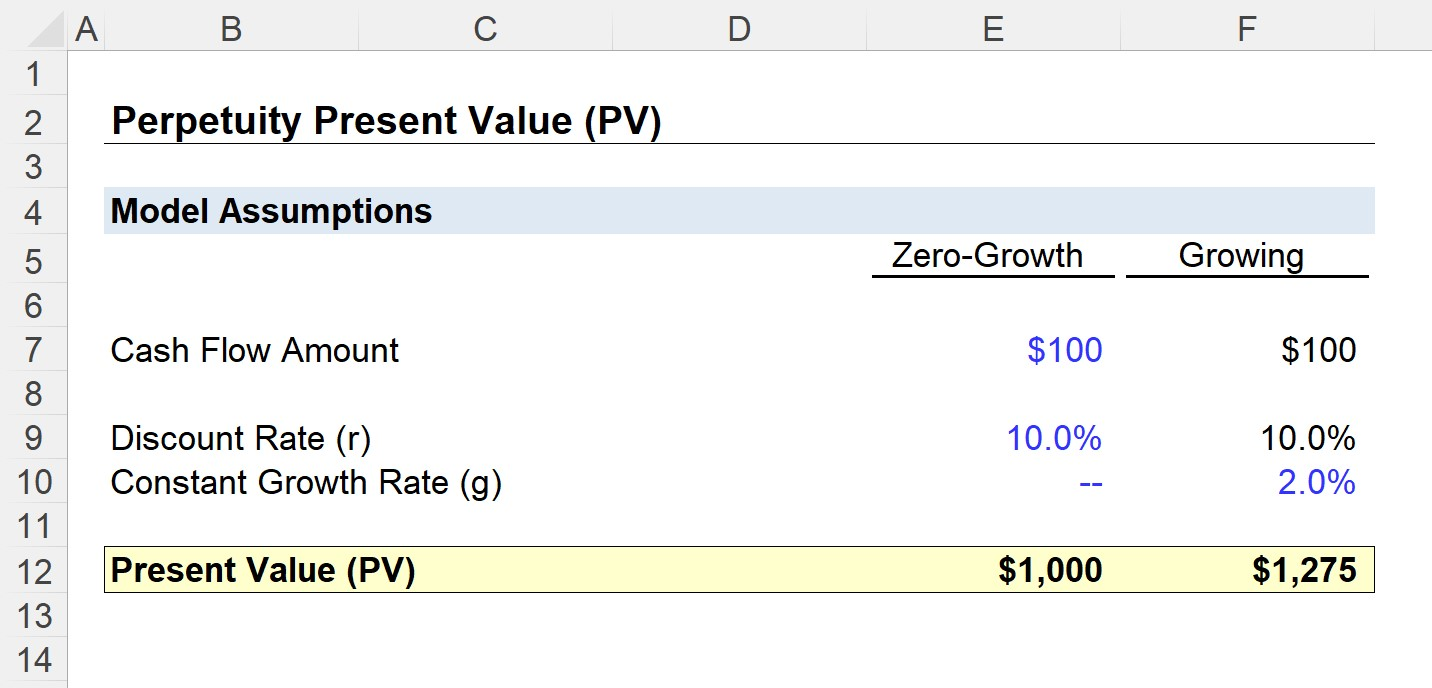Welcome to Wall Street Prep! Use code at checkout for 15% off.# Perpetuity

Guide to Understanding the Perpetuity Concept## How to Calculate Present Value of Perpetuity (PV)?

In a perpetuity, the series of cash flows received by the investor is expected to be received forever (i.e. a never-ending stream of cash flows).

For instance, if an investment comes with terms stating that a \$1,000 payment will be paid out at the end of each year with an indefinite end, this represents an example of a zero-growth perpetuity (i.e. the annual payout remains the same through the life of the investment).

Despite the cash flows theoretically lasting “forever,” the present value (PV) – i.e. the approximate valuation of the total potential stream of cash flows as of the current date – can still be calculated.

The “time value of money,” a fundamental concept in corporate finance, states that the further away from the date of when a cash flow payment is received, the greater the reduction in its value today.

As a result, the present value (PV) of the future cash flows of a perpetuity eventually reaches a point where the cash flow payments in the far future have a present value of zero.

## Perpetuity vs. Annuity: What is the Difference?

• Perpetuity → To reiterate, a perpetuity is a cash flow expected to continue forever with no ending date.
• Annuity → In contrast, an annuity comes with a pre-determined maturity date, which is when the final cash flow payment is received.

## Growing Perpetuity vs. Zero-Growth Perpetuity: What is the Difference?

In the prior example, the size of the cash flow (i.e. the \$1,000 annual payment) is kept constant throughout the entire duration of the perpetuity.

However, for growing perpetuities, there is a perpetual (or “continuous”) growth rate attached to the series of cash flows.

If we assume equal initial payment amounts, a growing perpetuity will thus be valued higher than one with zero-growth, all else being equal.

For example, if the investment stated that \$1,000 would be issued in the following year but at a 2% growth rate, then the annual cash flows would increase 2% year-over-year (YoY).

Since the cash flows increase each year, the growth rate helps offset the discount rate used to calculate the present value (PV).

## Perpetuity Formula

To calculate the present value (PV) of a perpetuity with zero growth, the cash flow amount is divided by the discount rate.

Present Value of Zero-Growth Perpetuity (PV) = Cash Flow ÷ Discount Rate

The discount rate is a function of the opportunity cost of capital – i.e. the rate of return that could be obtained from other investments with a similar risk profile.

For a growing perpetuity, the formula consists of dividing the cash flow amount expected to be received in the next year by the discount rate minus the constant growth rate.

Present Value of Growing Perpetuity (PV) = Year 1 Cash Flow ÷ (Discount Rate Growth Rate)

## Present Value of Perpetuity Calculator (PV)

We’ll now move to a modeling exercise, which you can access by filling out the form below.Submitting...

## 1. Present Value of Perpetuity Calculation Example

In our illustrative scenario, we will compare two perpetuities, sharing the following assumptions:

• Cash Flow Amount (Year 0) = \$100
• Discount Rate (r) = 10.0%

The difference between the two perpetuities is their respective growth rate assumptions:

• Zero Growth = 0.0% Growth Rate
• Growing = 2.0% Growth Rate

For the first zero growth perpetuity, the \$100 annual payment amount remains fixed, while the payment for the second perpetuity grows at 2.0% per year perpetually.

For the zero-growth perpetuity, we can calculate the present value (PV) by simply dividing the cash flow amount by the discount rate, resulting in a present value of \$1,000.

• Present Value (PV), Zero-Growth = \$100 Cash Flow ÷ 10.0% Discount Rate = \$1,000

If someone came to us and offered to sell the investment to us, we’d only proceed with investing if the purchase price is equal to or less than \$1,000.

Otherwise, the investment would not make much sense economically.

## 2. Present Value of Growing Perpetuity Calculation Example

Next, for the growing perpetuity, the first step is to grow the Year 0 cash flow amount by 2% once to arrive at the Year 1 cash flow amount.

• Year 1 Cash Flow = \$100 Year 0 Cash Flow × (1 + 2.0% Growth Rate)
• Year 1 Cash Flow = \$102

The denominator is equal to the discount rate subtracted by the growth rate.

• Present Value of Growing Perpetuity (PV) = \$102 ÷ (10.0% – 2.0%) = \$1,275

In conclusion, we can see the positive impact that growth has on the value of a perpetuity, as the present value (PV) of the growing perpetuity is \$275 more than the zero-growth perpetuity.Step-by-Step Online Course

### Everything You Need To Master Financial Modeling

Enroll in The Premium Package: Learn Financial Statement Modeling, DCF, M&A, LBO and Comps. The same training program used at top investment banks.

Inline Feedbacks